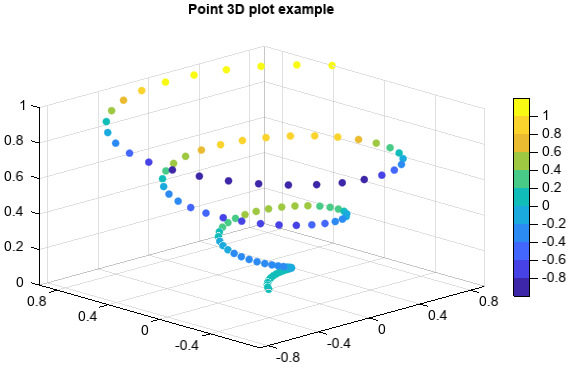# scatter3¶

Axes3DGL.scatter(x, y, z, s=8, c='b', marker='o', **kwargs):

Make a 3D scatter plot of x, y and z, where x, y and z are sequence like objects of the same lengths.

Parameters
• x – (array_like) Input x data.

• y – (array_like) Input y data.

• z – (array_like) Input z data.

• s – (int) Size of points.

• c – (Color) Color of the points. Or z vlaues.

• alpha – (int) The alpha blending value, between 0 (transparent) and 1 (opaque).

• marker – (string) Marker of the points.

• label – (string) Label of the points series.

• levels – (array_like) Optional. A list of floating point numbers indicating the level points to draw, in increasing order.

Returns

Point 3D graphics.

Example of 3D point plot

```z = linspace(0, 1, 100)
x = z * np.sin(20 * z)
y = z * np.cos(20 * z)
c = x + y

#Plot
scatter3(x, y, z, c=c)
colorbar(shrink=0.8)
title('Point 3D plot example')
```